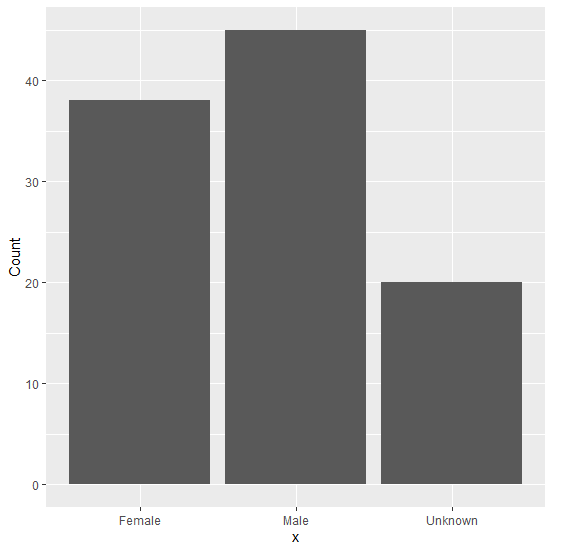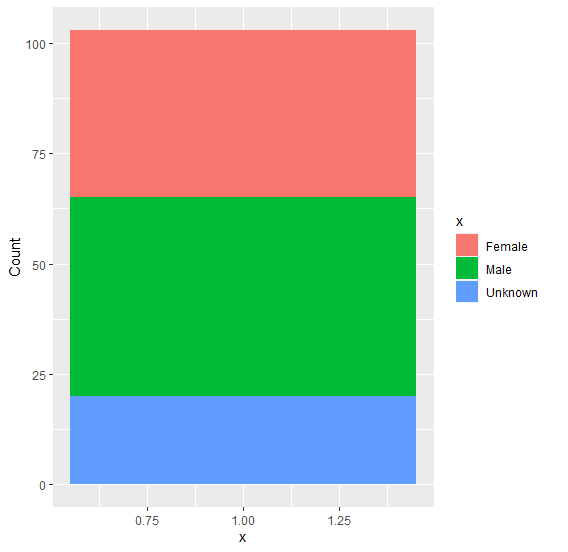# Create stacked bar plot for one categorical variable in an R dataframe.

To create stacked bar plot for one categorical variable in an R data frame, we can use ggplot function and geom_bar function of ggplot2 and provide 1 as the X variable inside aes.

For Example, if we have a data frame called df that contains a one categorical column say C and one numerical column Num then we can create the stacked bar plot by using the below command −

ggplot(df,aes(1,Num,fill=C))+geom_bar(stat="identity")

## Example

Following snippet creates a sample data frame −

x<-c("Male","Female","Unknown")
Count<-c(45,38,20)
df<-data.frame(x,Count)
df

The following dataframe is created

       x  Count
1    Male   45
2  Female   38
3 Unknown   20

To load ggplot2 package and create bar plot for values in df on the above created data frame, add the following code to the above snippet −

x<-c("Male","Female","Unknown")
Count<-c(45,38,20)
df<-data.frame(x,Count)
library(ggplot2)
ggplot(df,aes(x,Count))+geom_bar(stat="identity")

## Output

If you execute all the above given snippets as a single program, it generates the following Output −To create stacked bar plot for values in df on the above created data frame, add the following code to the above snippet −

x<-c("Male","Female","Unknown")
Count<-c(45,38,20)
df<-data.frame(x,Count)
library(ggplot2)
ggplot(df,aes(1,Count,fill=x))+geom_bar(stat="identity")

## Output

If you execute all the above given snippets as a single program, it generates the following Output −